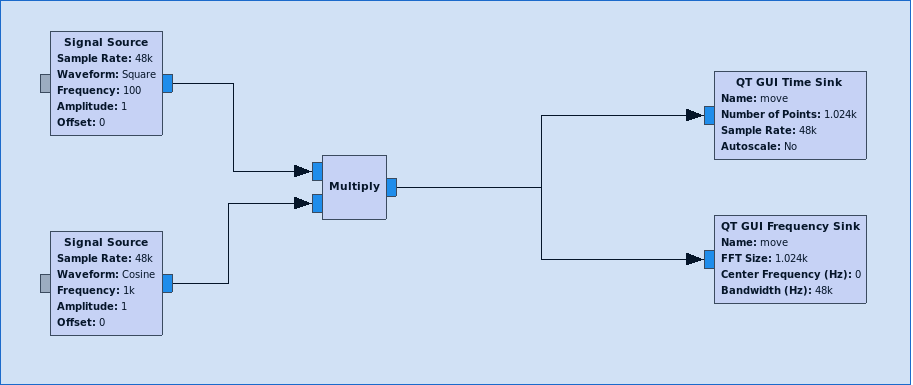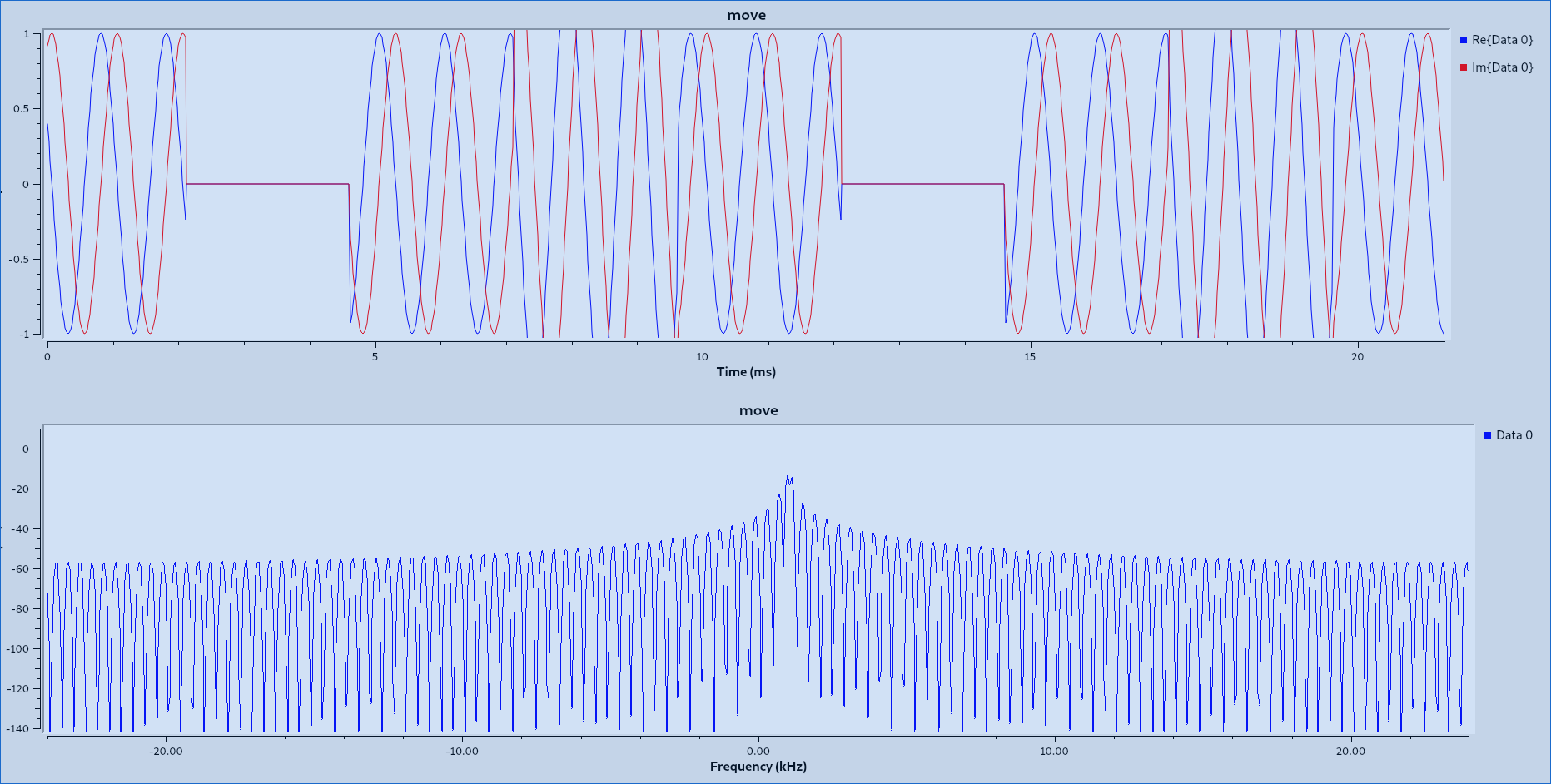# How to shift frequency with sine signal?

cosine signal can make square signal shift frequency.If I have only sine signal source,how to make square signal shift frequency?

• You need to understand real signal versus complex spectrum. Complex multiplication of a (complex; I and Q components) input signal with Sine/Cosine as shift frequency results in a complex output signal. The problem is the generation of a square wave as complex signal: limited spectrum. Wait for a better explanation.
– user16925
Nov 5, 2021 at 12:31
• Note that a sine wave has no harmonics; a square wave has an infinite number of harmonics. Nov 5, 2021 at 18:14